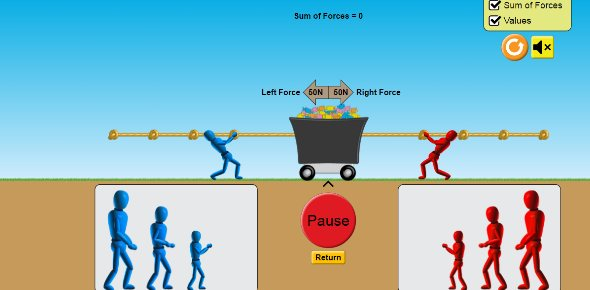Force And Motion Review

19 Questions | Total Attempts: 199Settings• 1.
An unbalanced force will cause an object to ____________ .
• A.

Remain at rest

• B.

Remain in uniform motion

• C.

Accelerate

• D.

Travel through time

• 2.
If an elephant steps on a flea which applies the greater force on the other?
• A.

The flea

• B.

The elephant

• C.

They apply equal forces on each other

• D.

Neither applies a force on the other

• 3.
Kate throws a snowball up into the air.  What forces are acting on the snowball after it leaves Kate's mitten?
• A.

The force from Kate's arm.

• B.

The force from Kate's arm and gravity.

• C.

Gravity and the normal force.

• D.

Just gravity.

• 4.
The force that opposes gravity and keeps us from falling towards the center of the earth is called the __________ .
• A.

Gravitational force

• B.

Normal force

• C.

Newtonian force

• D.

Friction force

• 5.
Check all the options below that are examples of friction.
• A.

Brakes stopping a car

• B.

A kid riding a skateboard

• C.

Tread on boots helping you not slip on ice

• D.

A book at rest on a table

• 6.
Applying a constant force to an object will cause it to move at uniform motion.
• A.

True

• B.

False

• 7.
The English Scientist _______________ first presented his three laws of motion in his 1686 book Philosophiae Naturalis Principia Mathematica.
• A.

Albert Einstein

• B.

Galileo Galiei

• C.

Isaac Ring

• D.

Isaac Newton

• 8.
Kelly is designing an experiment to see if listening to music while studying helps with assessment scores.  She gets 10 of her friends to help in her experiment and divides them into two different test groups.  5 of them study a set of vocabulary words while listening to musics and 5 of them study without listening to music. She then gives them all the same test to see which group does better on the vocabulary words, the "music group" or the "no music group". What is Kelly's independent variable?
• A.

The different test groups

• B.

The scores they get on the test

• C.

The vocabulary words they have to study

• D.

The test they take

• 9.
When the mass of an object increases the amount of acceleration ___________ .
• A.

Increases

• B.

Decreases

• C.

Remains the same

• 10.
If the forces on an object are balanced what type of motion does this cause?
• A.

Acceleration

• B.

Uniform Motion

• C.

No motion/at rest

• D.

Uniform motion or no motion

• 11.
A coaster car runs into a desk, stopping the car. The desk is unharmed. Which applies the larger force to the other?
• A.

The desk

• B.

The car

• C.

Neither applies a force on the other

• D.

They apply equal size forces on each other

• 12.
Which experienced the greater net force? The coaster car or the desk?
• A.

The desk

• B.

The car

• C.

The net force was equal between the two objects

• D.

Option 4

• 13.
What is Kelly's dependent variable?
• A.

The different test groups

• B.

The scores they get on the test

• C.

The vocabulary words they have to study

• D.

The test they take

• 14.
What would some of Kelly's control variables be in her experiment? Check all that apply
• A.

The vocabulary words they study

• B.

The different test groups

• C.

The test they take

• D.

The scores they get on the test

• 15.
Force and ___________ have a positive relationship.
• A.

Gravity

• B.

Mass

• C.

Acceleration

• D.

Net force

• 16.
Acceleration and ___________ have a negative relationship.
• A.

Gravity

• B.

Mass

• C.

Acceleration

• D.

Net force

• 17.
This interaction between the flea and the elephant is an example of _______________.
• A.

Newton's 1st Law of Motion

• B.

Newton's 2nd Law of Motion

• C.

Newton's 3rd Law of Motion

• 18.
This relationship between force, mass and acceleration is stated in  _______________.
• A.

Newton's 1st Law of Motion

• B.

Newton's 2nd Law of Motion

• C.

Newton's 3rd Law of Motion

• 19.
A book at rest on a table is experiencing _______________.
• A.

Friction

• B.

Balanced forces

• C.

Unbalanced forces

• D.

Net force

Related TopicsBack to top